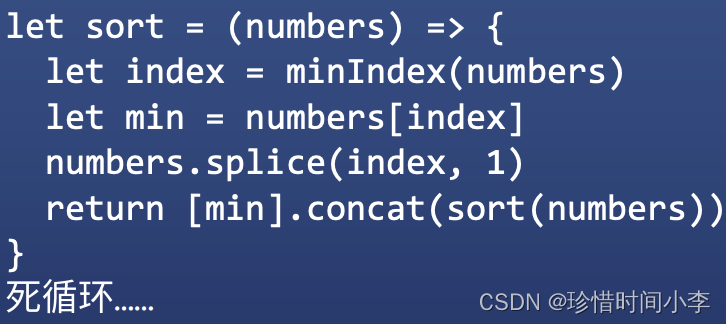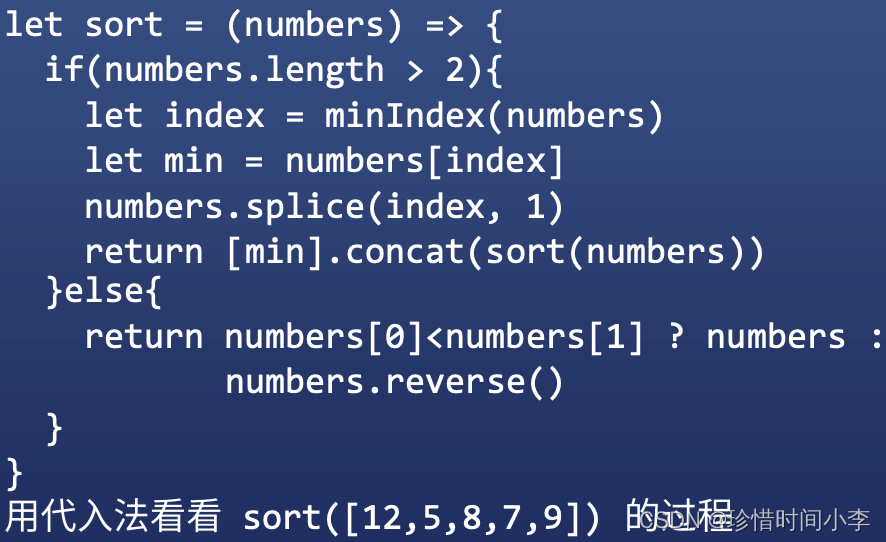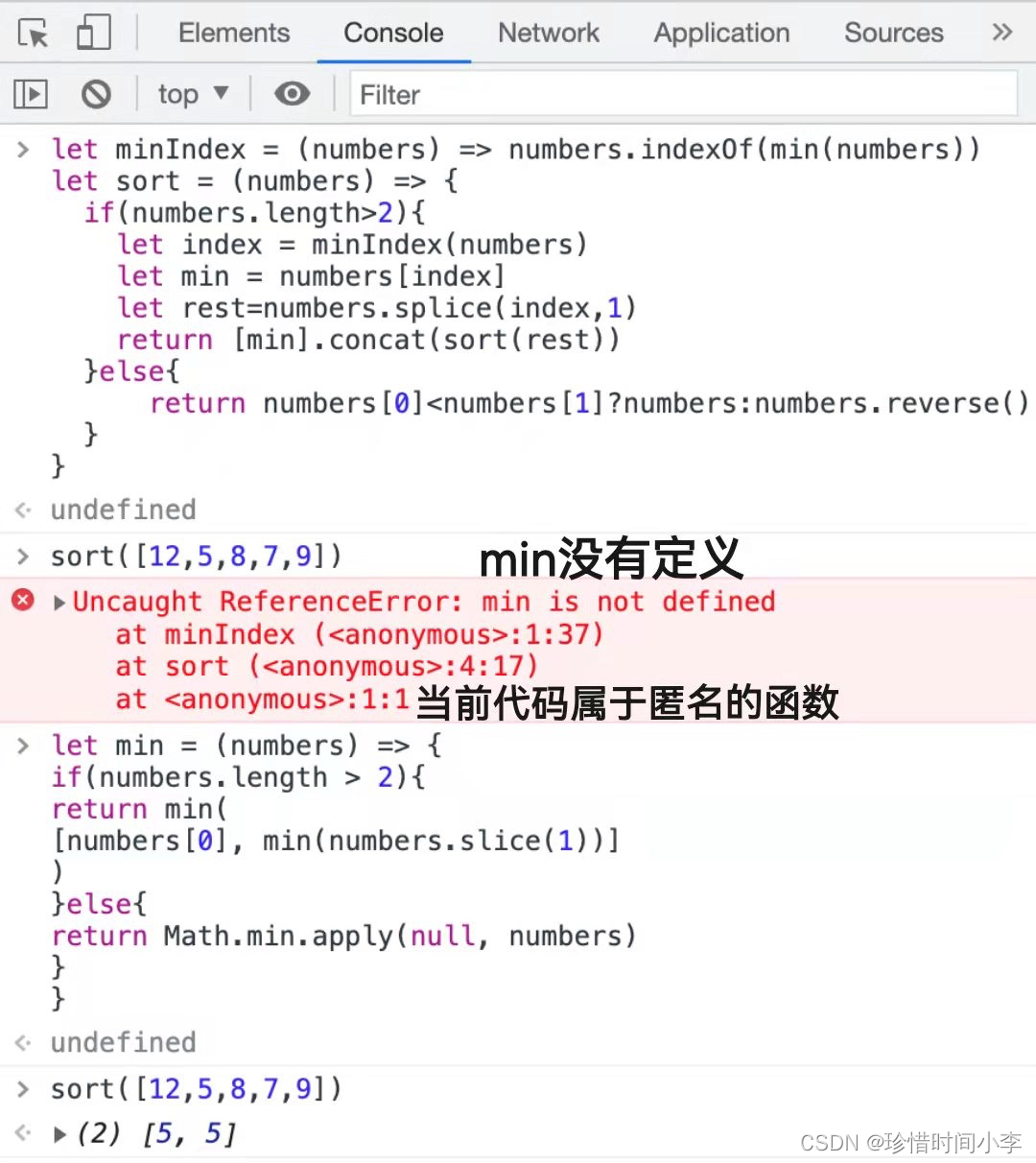# 排序算法(上)

2020-10-16

析构赋值:把结构拆开，依次复制
let minOf2=([a,b]) => a < b ? a : b
//调用
minOf2([1,2]) //小白调用法
minOf2.call(null,[1,2]) //高手调用法


JS内置了Math.min
Math.min(1,2)
Math.min.call(null,1,2)
Math.min.apply(null,[1,2])

1.numbers.slice(1) 选中从下标1开始的所有数据
2.Math.min.apply(null,numbers)
apply就是把numbers一个个展开调用。call就是把numbers作为一项。

var arr = [1,3,6,8,2,10];
var minNum = Math.min.apply(null,arr);
console.log(minNum);  //1


Math.min 可以实现得到数组中最小的一项
Math.min.apply(null,arr)其中第一个参数null，这个是因为没有对象去调用这个方法，所以直接传递null过去。同理,Math.max.apply可以获得数组里面最大的值。

### 实现sort排序(将正整数数组从小到大排序)

#### 递归思路

let minIndex = (numbers) => numbers.indexOf(min(numbers))
//取巧:如果有2个最小数，只会返回第1个最小数的下标。

let sort3 = (numbers) => {
//获取最小数字的下标
let index = minIndex(numbers) //minIndex函数
let min = numbers[index]
//获取最小数下标后再将其删掉,返回被保留的数字
numbers.splice(index, 1)
return [min].concat(sort2(numders))
}


1.JS中concat用于2个数组的相加，拼成一个数组
2.sort2(numders)得到的是排好序的2个数
3.numbers.indexOf求出某个数字的下标要理解递归，必须用代入法

let minIndex = (numbers) => numbers.indexOf(min(numbers))
let sort = (numbers) => {
if(numbers.length>2){
let index = minIndex(numbers)
let min = numbers[index]
let rest=numbers.splice(index,1)
return [min].concat(sort(rest))
}else{
return numbers<numbers?numbers:numbers.reverse()
}
}


1.<anonymous>，当前代码属于匿名的函数
2.at sort，调用了sort1.求最小值: 2个数、3个数、N个数
2.排序: 2个数、3个数、N个数

1.请写一个min函数，要求min(numbers)能返回数组numbers中的最小数字。

let min = (numbers) => {
if(numbers.length > 2){
return min([numbers, min(numbers.slice(1))])
}else{
return Math.min.apply(null, numbers)
}
}


2.请写出一个sort函数，要求sort(numbers)能返回一个把numbers 从小到大排列的数组

let minIndex = (numbers) => numbers.indexOf(min(numbers))
let sort = (numbers) => {
if(numbers.length > 2){
let index = minIndex(numbers)
let min = numbers[index]
numbers.splice(index, 1)
return [min].concat(sort(numbers))
}else{
return numbers<numbers ? numbers : numbers.reverse()
}
}
sort([21,8,44,92])